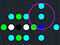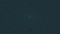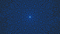# In math, there can be more complexity without complex numbers

As you might already know, a “complex number” is a number with both a nonzero “real” part and a nonzero “imaginary” part.

Or we could also say all numbers are complex, it’s just that the purely real numbers have 0 for an imaginary part, and technically that’s correct.

Generally though, “complex” means nonzero imaginary part. One might think that complex numbers make things more complicated. Sometimes, though, things are a lot more complex without complex numbers.

I’ve been writing a lot about my Java program that draws diagrams of prime numbers on the complex plane. But just prime numbers on the real number line are also interesting.

The thing though is that, for example, prime numbers of the form a + b√−2 are neatly spaced throughout the complex plane, where by contrast prime numbers of the form a + b√2 are all crowded together on the real number line.

Sure, it’s as easy to locate a number like 3 + √2 as it is to locate a number like 3 + √−2. But the question of what those numbers’ neighbors are in their particular domain gives radically different answers.

Just a bit of terminology before we go any further: all numbers of the form a + b√−2 form an “imaginary” quadratic integer “ring” usually notated as Z[√−2].

Likewise, all numbers of the form a + b√2 form a “real” quadratic integer “ring” usually notated as Z[√2].

All rings are “closed under” addition and multiplication, meaning that if we add or multiply any two numbers from the same ring, the result is also in the same ring.

To find the numbers of the form a + b√−2 that are within a √3 radius of 3 + √−2, we just have to iterate a from 2 to 4 and b from 0 to 2. And so we get, reading “clockwise” from “12 o’clock”: 3 + 2√−2, 4+ 2√−2, 4+ √−2, 4, 3, 2, 2 + √−2 and 2 + 2√−2. Eight numbers total.

Actually, four of those numbers are exactly √3 away from 3 + √−2, so if we understand “within” to exclude those numbers, then 3 + √−2 only has four neighbors within a √3 radius.

Under either interpretation, there is a finite set of neighboring numbers within the specified radius. For any finite area of the complex plane, there is a finite set of numbers from a given imaginary quadratic ring that are in that area.

Here is a diagram:Some prime numbers in Z[√−2] as cyan dots. The black dot is 0, the green dots on the same horizontal line as 0 are −2 and 2. The dark blue dot is 3 and the cyan dot above it is 3 + √−2. The magenta circle is centered on 3 + √−2 and has a diameter of 2√3.

To examine the seemingly similar question of how many numbers of the form a + b√2 are within a given radius of 3 + √2, we only need to look at the real number line. Since a and b are purely real rational integers, and √2 is purely real (though irrational), the number a + b√2 is sure to also be purely real.

So no complex numbers, we can focus our attention on the real number line. This should be easier, right?

Just in case, though, let’s narrow the radius from √3 to 1/2. Since 3 + √2 is approximately 4.4142, we’re looking for numbers between roughly 3.9142 and roughly 4.9142.

It might feel wrong to not have much numerical precision, but, as it will turn out, we don’t need a lot of numerical precision to come to the right conclusion.

The first number that I can think of as a potential neighbor is 6 − √2, which is roughly 4.5858. Since this is roughly 1/5 more than 3 + √2, it falls well within the specified radius of 1/2.

The next number I’m thinking of is 7 − 2√2, roughly 4.17158. And then there is 9 − 3√2, roughly 4.7574; 10 − 4√2, roughly 4.3431; 11 − 5√2 is about 0.0147 shy of the radius but still within it; 13 − 6√2 is roughly 4.5147; etc.

The only reason we can’t keep this going forever is that eventually a and b will go beyond the range our computers can handle. However, this is enough to come to the conclusion that 3 + √2 has infinitely many neighbors in this ring within a radius of 1/2.

And that’s a conclusion we reached looking only at pairs of a and b with a > 3 and b negative. If we look at a < 3 and positive b, there is a whole other infinity of neighbors. And I just realized I skipped over 4 (which corresponds to b = 0).

The following diagram of Z[√−2] was generated by a Java program that I wrote:Some prime numbers in Z[√−2] as cyan dots. This is at 2 pixels per unit interval if viewed at full resolution.

The program is a work in progress. An executable is available from my GitHub page, along with the source code and unit tests in nearby directories.

If I’m certain that I wrote the program correctly, then I can also be certain that this diagram shows every prime number in Z[√−2] with real part ranging from −320 to 320 and imaginary part ranging from −180√−2 to 180√−2.

With more pixels per unit interval, the diagram also shows grid intersections for numbers that in the given domain that are not primes, units or 0.

Just within 1/2 of 3 + √2, which is prime with a norm of 7, there are numbers with norms of 41, 63, 68, 71, 97, etc. A diagram of prime numbers in Z[√2] couldn’t show every prime number in a given range, much less every number.

The earlier diagram was also generated by my Java program, but I cropped it and added the magenta circle in Adobe Photoshop Elements. This other diagram of Z[√2], on the other hand, was created entirely in Photoshop Elements:Some prime numbers in Z[√2] as cyan lines. I can’t vouch for the mathematical accuracy of this diagram.

To get around the difficulty of infinitely many numbers in a given range, like −320 to 320, I decided I would only concern myself with numbers having norms close to 0, like −43 and 25.

I would not worry about numbers like 32771 − 23170√2, which is prime with a norm of 240641 and has a numerical value of roughly 3.6718.

My program can generate diagrams in seconds if not milliseconds. It takes me hours if not days to create a diagram by clicking around in Photoshop, and the process is error-prone.

Logically I should expand my program so that in addition to diagrams of number domains like Z[√−2] that have complex numbers it is also able to draw diagrams of number domains like Z[√2] that don’t have complex numbers.

In this article I’m not going to go too in depth on the nuts and bolts of the Java program as I do in other articles. As much as I can, I’ll try to explain those concepts as simply as I can.

As I’ve explained in other articles, I was initially skeptical of automated testing. But since I’m not working in artificial intelligence, I don’t have to worry about the computer trying to fool me.

As a purely practical matter, even a moderately obsolete computer can generate more data in a second than I can check in a day. So I rely on JUnit, a widely available testing framework for Java, to check my program.

One of the first things I had JUnit check on this program is that it can correctly add and multiply imaginary quadratic integers. Then I had it check that my program can correctly factorize imaginary quadratic integers.

Even without preparing extensive multiplication tables, there are plenty of ways I can write tests for the prime factorization function, as long as I’m satisfied the basic arithmetic functions pass their corresponding tests.

For one thing, if my program says, for example, that a particular number is the product of three numbers, then multiplying those three numbers should give the original number as a result.

One caveat, however, is that the prime factorization function only works on unique factorization domains (UFDs). If you try to use it on a number that’s not from an UFD, it throws an exception.

However, the exception provides a function to try to factorize the number anyway, if not as a product of primes, then as a product of irreducible numbers.

To indicate the presence of irreducible but not prime factors, the attempted factorization function returns a list with twice as many extraneous instances of −1 as there are irreducible non-prime factors.

For example, the program might give the factorization of 25 + √−5 as −1 × −1 × −1 × −1 × −1 × (√−5)(1 + √−5)(4 + √−5). Likewise it might give the factorization of 6 in Z[√−5] as −1 × −1 × −1 × −1 × 2 × 3.

In both cases, the extraneous (−1)s let us know a different factorization into irreducibles may be possible, such as the famous (1 − √−5)(1 + √−5) = 6. In the 25 + √−5 example, only one −1 is needed, the rest are extraneous.

I got my program to work with imaginary quadratic integers. For the most part anyway; I recently discovered a couple of examples of numbers for which the program gives the wrong results.

Eventually I will have to figure out what those imaginary quadratic integers have in common, so that I can correct the program.

I think the problem most likely is a silly oversight on my part, not the result of some exotic property of a few imaginary quadratic integers. The program does give correct results for lots of other numbers I’ve tested.

Thinking I had things all set for imaginary quadratic integers, I moved on to real quadratic integers. I wouldn’t be dealing with complex numbers, but that certainly didn’t mean I wouldn’t run into complicated problems.

And even though I was aware of some aspects of real quadratic integers that could turn out to be problematic, those aspects still caused me problems just the same.

Once I was satisfied with the basic arithmetic functions on real quadratic integers, I started work on programming the factorization function of numbers in real quadratic rings that are not UFDs.

I would expect the factorization of 6 in Z[√10] to be given as as −1 × −1 × −1 × −1 × 2 × 3, since (4 − √10)(4 + √10) is one possible alternate factorization.

Another example to use in the tests is −40 − 11√10, roughly −74.785, with a norm of 390. I came up with that number as −1 × (5 + √10)(6 + √10).

My program erroneously factorizes it as −1 × −1 × −1 × −1 ×(√10)(11 + 4√10). That would be correct if it had one more instance of −1.

So when JUnit runs through that factorization, it comes up with 40 + 11√10, which does not match the expected value of −40 − 11√10. The test fails and I get a yellow triangle instead of a green circle in the test results window.

It’s actually the same problem I’m having with 25 + √−5. I still think this will just be a matter of sitting down to figure it out.

I had a far more serious problem before that test failure: trying to run the tests, JUnit would just get stuck on a real quadratic integer test.

A failing test is one thing, it’s a step towards figuring out the correct algorithm. A stuck test runner, on the other hand, could be the result of the kind of novice mistake I ought to know better about by now.

The algorithm is nothing sophisticated, just plain old trial division. Clearly −40 − 11√10 is not divisible by 2, 3, 5, 7, 11 or 13. It’s not divisible by 1 + √10 either, nor by 2 + √10.

These divisions trigger `NotDivisibleException`, which the factorization function catches and then moves on to the next potential divisor. So far so good.

The problem arises with 3 + √10, which is the fundamental unit of Z[√10]. We call it “fundamental” because there are infinitely many units in Z[√10] and through this unit, which is the smallest unit that is greater than 1, we can find all other units of Z[√10].

I was aware of this when I started working on the program, and I should have foreseen that it could arise as a problem as I worked to expand the program to work with real quadratic integer rings.

We see that −40 − 11√10 divided by 3 + √10 is −7 + √10, which has a norm of 39. And −7 + √10 divided by 3 + √10 is 31 − 10√10, which has a norm of −39. And 31 − 10√10 divided by 3 + √10 is −193 + 61√10, which has a norm of 39. And −193 + 61√10 divided by…

Theoretically we could keep this up forever. In practice, JUnit could only keep this up until one of four things happened:

• The Java Virtual Machine runs out of memory.
• JUnit execution is forcefully terminated.
• The program hosting JUnit is forcefully terminated.
• The computer is abruptly turned off.

It’s certainly possible that the numbers get beyond what my program can represent, leading to an incorrect division, but the program would still not move on to trying the next potential divisor, in this case 4 + √10.

In the example, −193 + 61√10 is roughly −0.10106273, while 1189 − 376√10 is roughly −0.01640022. The estimated numerical value is getting close to 0, but the numbers a and b of a + b√10 could soon exceed 2³¹ − 1, which are the largest positive values of a and b my program can handle.

If I had put in some robust overflow checking in the basic arithmetic functions, maybe this would trigger an exception and finally put a stop to this runaway division.

But instead of an overflow exception, the division function simply returns an incorrect, though predictable, value. It doesn’t actually matter what that number is, because as long as it’s a number in Z[√10], it’s divisible by 3 + √10.

And so the runaway division keeps going, unless something external to it stops it.

This had me completely puzzled. I went over the factorization function line by line and couldn’t see what the problem was. I added a bunch of “console logs” to print out what the test divisor was.

Then I finally saw the cause of the runaway division:

I felt so silly when I saw this. I knew that Z[√10] has infinitely many units and I knew that 3 + √10 is its fundamental unit. Knowing all that, it still did not occur to me I would have to watch out for it in writing my program.

The fix was easy: have the function check that the potential test divisor is not a unit by checking the absolute value of its norm.

This is just not an issue with imaginary quadratic rings. Almost all of them have exactly two units: −1 and 1; the most famous imaginary quadratic ring has four units and the second most famous imaginary quadratic ring has just six units.

Also, negative norms are impossible in imaginary quadratic rings (in the context of my program, a negative norm for an imaginary quadratic integer might indicate an overflow).

So if I did have to watch out for units in an imaginary quadratic integer ring, it would be enough to check that the norm of an imaginary quadratic integer is not 1.

Some real quadratic rings don’t have units of norm −1, but Z[√10] does: the norm of 3 + √10 is −1. The same is also true of units that are odd powers of 3 + √10.

Of course it’s possible to bring the headaches of real quadratic rings to the complex plane. One way is with rings of algebraic degree 4. I can’t vouch for the mathematical accuracy of the following diagram, and in any case I know it to be incomplete.Output of the first draft of my program to draw a diagram of primes in the ring of algebraic integers of Q(√2/2 + √−2/2).

Maybe this diagram would be more accurate if it just had a solid cyan background with just a few select dots over it. I still have a lot of math to work out for that one, as well as the problems with quadratic integers.

is a composer and photographer from Detroit, Michigan. He has been working on a Java program to display certain mathematical diagrams.

## More from Alonso Del Arte

is a composer and photographer from Detroit, Michigan. He has been working on a Java program to display certain mathematical diagrams.